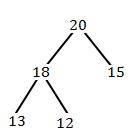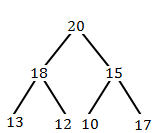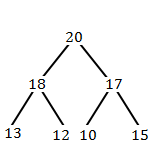# UGC NET COMPUTER SCIENCE SOLVED PAPERS 2014-16 - UGC NET Computer Science Paper 3 August 2016

>>>>>>>>UGC NET Computer Science Paper 3 August 2016

• A

14875• B

21000• C

9375• D

11875• A

46• B

41• C

43• D

48• Option : B
• Explanation :
Given hash function: h(K) = floor (m (K*A mod 1)) where A = ( √(5) – 1)/2
h(123456) = floor(10000 * (123456 * (√5 − 1) / 2) mod 1)
= floor(10000 * (76300.004115 mod 1)
= floor(10000 * (.004115))
= 41.15
= 41
So, option (B) is correct.

• A

4n2• B

n• C

4n - 4• D

2n - 2• A

20, 18, 17, 15, 13, 12, 10• B

20, 18, 17, 12, 13, 10, 15• C

20, 18, 17, 10, 12, 13, 15• D

20, 18, 17, 13, 12, 10, 15• Option : D
• Explanation :
Initially we have:When we insert 10 and 17:We have to maintain max-heap, so:The level-order traversal of the heap after the insertion of the element is 20, 18, 17, 13, 12, 10, 15 So, option (D) is correct.

• A

O (k (n + d))• B

O (d (n + k))• C

O ((n + k) lg d)• D

O ((n + d) lg k)• Option : B
• Explanation :
If there are n integers to sort, each integer has d digits, and each digit is in the set {1, 2, ..., k}, radix sort can sort the numbers in O(d (n + k)). For more information Refer:Radix Sort Option (B) is correct.